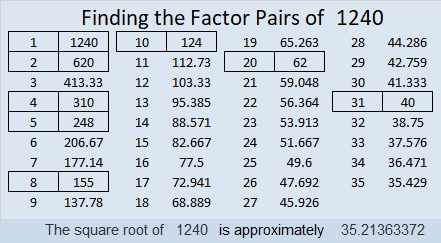# 1240 is a Square Pyramidal Number

1240 is the 15th square pyramidal number because
1² + 2² + 3² + 4² + 5² + 6² + 7² + 8² + 9² + 10² + 11² + 12² + 13² + 14² + 15² = 1240

We can know that 1240 is the 15th square pyramidal number because
15(15 + 1)(2·15 + 1)/6
= 15(16)(31)/6
= (5)(8)(31)
= (40)(31)
= 1240Here are some more facts about the number 1240:

• 1240 is a composite number.
• Prime factorization: 1240 = 2 × 2 × 2 × 5 × 31, which can be written 1240 = 2³ × 5 × 31
• The exponents in the prime factorization are 3, 1, and 1. Adding one to each and multiplying we get (3 + 1)(1 + 1)(1 + 1) = 4 × 2 × 2 = 16. Therefore 1240 has exactly 16 factors.
• Factors of 1240: 1, 2, 4, 5, 8, 10, 20, 31, 40, 62, 124, 155, 248, 310, 620, 1240
• Factor pairs: 1240 = 1 × 1240, 2 × 620, 4 × 310, 5 × 248, 8 × 155, 10 × 124, 20 × 62, or 31 × 40
• Taking the factor pair with the largest square number factor, we get √1240 = (√4)(√310) = 2√310 ≈ 35.213631240 is the hypotenuse of a Pythagorean triple:
744-992-1240 which is (3-4-5) times 248

This site uses Akismet to reduce spam. Learn how your comment data is processed.